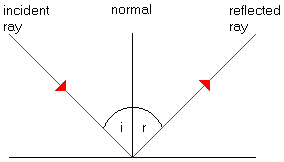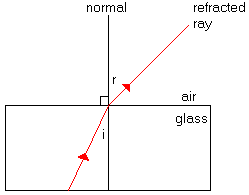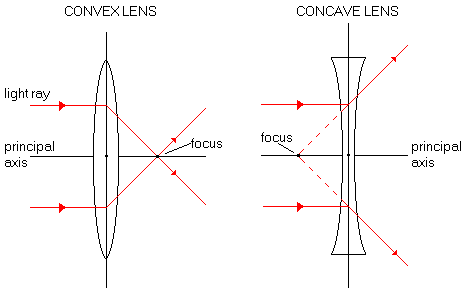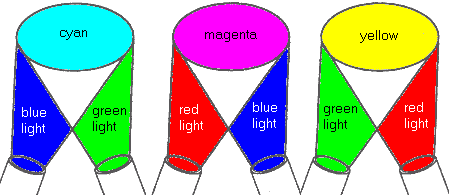# Light and Optics

## The Nature of Light

• Light is both waves and particles called photons.
• Some light is visible (i.e. red, orange, yellow, green, blue, indigo and violet) and some is invisible (e.g. infrared, ultraviolet, gamma rays, X-rays).
• Light travels in straight lines.
• The speed of light in a vacuum is 300 000 000 m/s. Light travels more slowly in more dense media such as glass and water.

## Reflection• Light travels in straight lines.
• Angle of Incidence (i) = Angle of Reflection (r)
• The object in front of a mirror is the same distance as the image is behind the mirror.

## Refraction• Refraction is the bending of light as it travels from one medium to another (e.g. air to glass).
• When travelling from a less dense to a more dense medium (e.g. air to water), light rays refract closer to the normal, and the angle of incidence is more than the angle of refraction.
• When travelling from a more dense to a less dense medium (e.g. water to air), light rays refract away from the normal, and the angle of incidence is less than the angle of refraction.

## Prisms

• A prism is a transparent object with flat surfaces that is used to separate the visible light spectrum into its colours by refraction.

## Critical Angle

• The critical angle is the angle of incidence that gives an angle of refraction of 90° with the normal and goes along the surface.
• When light rays travel at the critical angle through an object such as optical fibre, they are totally internally reflected.

## Images

• Images can be:
1. Real (able to be focused on a screen) or Virtual (cannot be focused on a screen)
2. Magnified (larger than object), Diminished (smaller than object) or Same Size
3. Erect (upright) or Inverted (upside down)

## Lenses• There are 2 main types of lenses - convex and concave.
• Convex lenses are converging lenses that focus the light rays to a point.
• Concave lenses are diverging lenses that spread the light rays.

## The Colour of Objects

• Objects have the colour that is reflected back to our eyes. Other light colours are absorbed by the object. For example, green plants reflect green light and absorb other coloured wavelengths for photosynthesis.
• If the colour of light shining on an object is not the same as the colour of the object, then the object will appear a completely different colour. For example, if blue light is shone on a red apple, the apple will appear black.
• Mixing paint colours is the opposite of mixing light colours. In the diagram below, for example, red and green light from spotlights make a yellow light.